# Chomskys Spell Checker Cohan Sujay Carlos Aiaioo Labs

• Slides: 64
Download presentation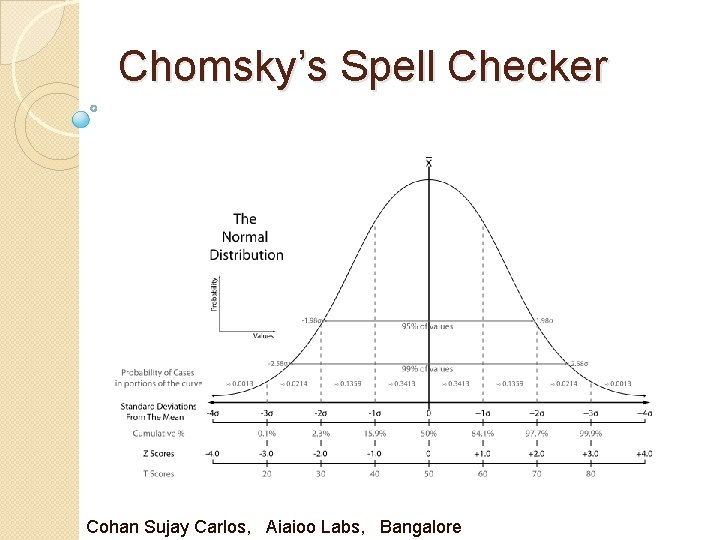Chomsky’s Spell Checker Cohan Sujay Carlos, Aiaioo Labs, Bangalore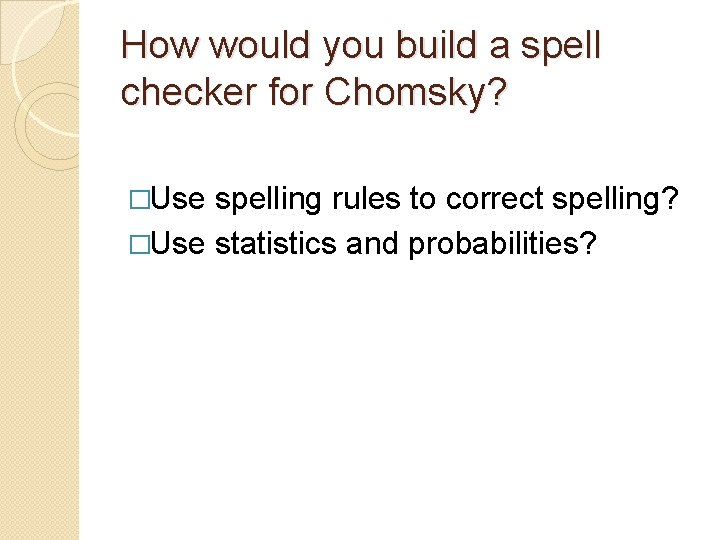How would you build a spell checker for Chomsky? �Use spelling rules to correct spelling? �Use statistics and probabilities?Chomsky Derided researchers in machine learning who use purely statistical methods to produce behaviour that mimics something in the world, but who don't try to understand the meaning of that behaviour.Chomsky Compared such researchers to scientists who might study the dance made by a bee returning to the hive, and who could produce a statistically based simulation of such a dance without attempting to understand why the behaved that way.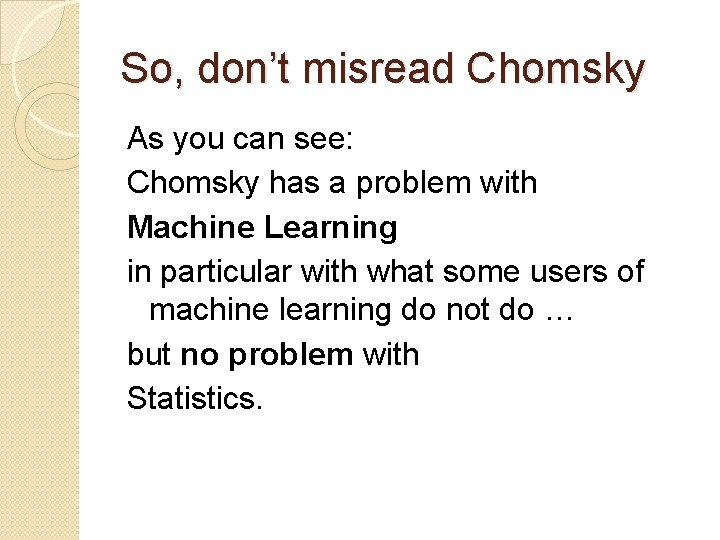So, don’t misread Chomsky As you can see: Chomsky has a problem with Machine Learning in particular with what some users of machine learning do not do … but no problem with Statistics.Uses of Statistics in Linguistics �How can statistics be useful? �Can probabilities be useful?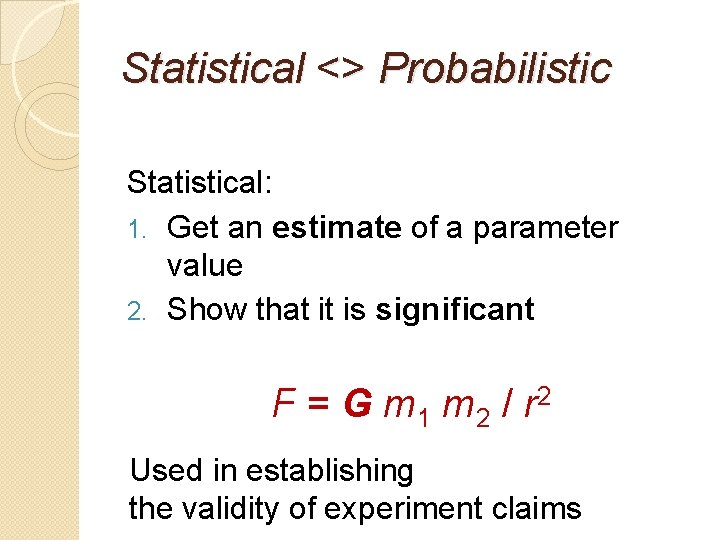Statistical <> Probabilistic Statistical: 1. Get an estimate of a parameter value 2. Show that it is significant F = G m 1 m 2 / r 2 Used in establishing the validity of experiment claims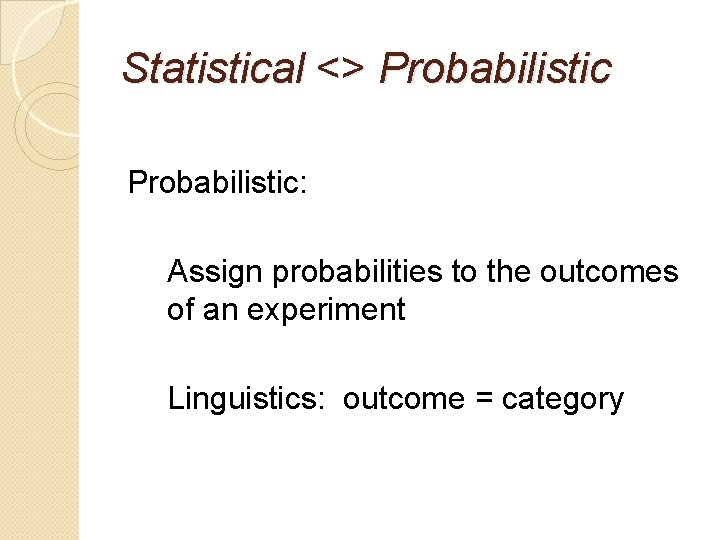Statistical <> Probabilistic: Assign probabilities to the outcomes of an experiment Linguistics: outcome = category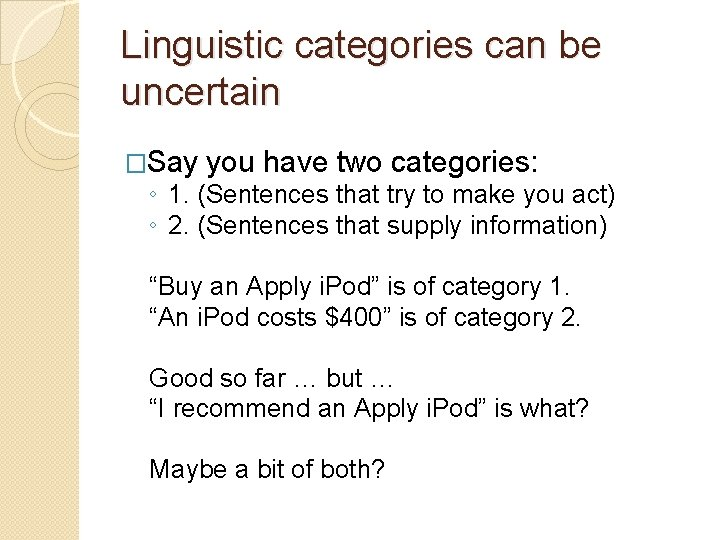Linguistic categories can be uncertain �Say you have two categories: ◦ 1. (Sentences that try to make you act) ◦ 2. (Sentences that supply information) “Buy an Apply i. Pod” is of category 1. “An i. Pod costs \$400” is of category 2. Good so far … but … “I recommend an Apply i. Pod” is what? Maybe a bit of both?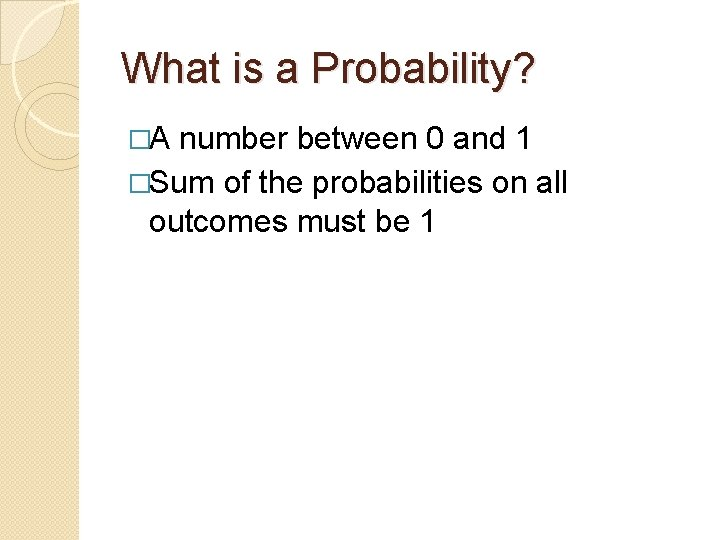What is a Probability? �A number between 0 and 1 �Sum of the probabilities on all outcomes must be 1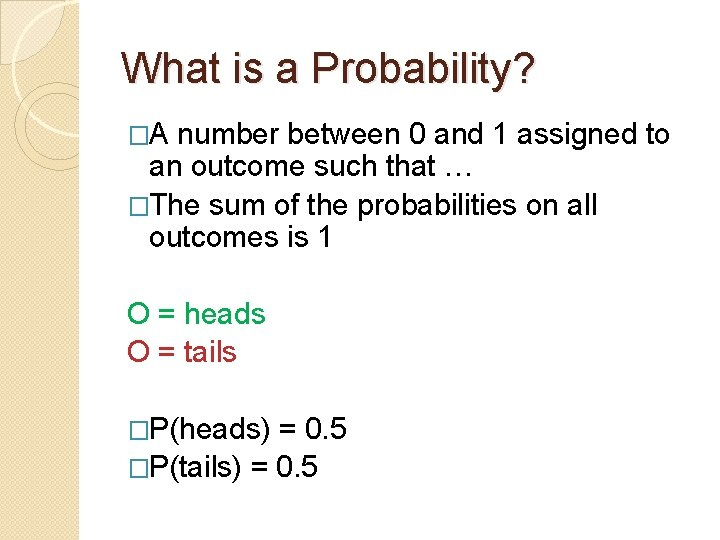What is a Probability? �A number between 0 and 1 assigned to an outcome such that … �The sum of the probabilities on all outcomes is 1 O = heads O = tails �P(heads) = 0. 5 �P(tails) = 0. 5Categorization Problem : Spelling 1. 2. 3. 4. 5. 6. Field Wield Shield Deceive Receive CeilingRule-based Approach “I before E except after C” -- an example of a linguistic insightProbabilistic Statistical Model: �Count the occurrences of ‘ie’ and ‘ei’ and ‘cie’ and ‘cei’ in a large corpus P(IE) = 0. 0177 P(EI) = 0. 0046 P(CIE) = 0. 0014 P(CEI) = 0. 0005Words where ie occur after c �science �society �ancient �speciesCourtesy Peter Norvig’s article on Chomsky http: //norvig. com/chomsky. html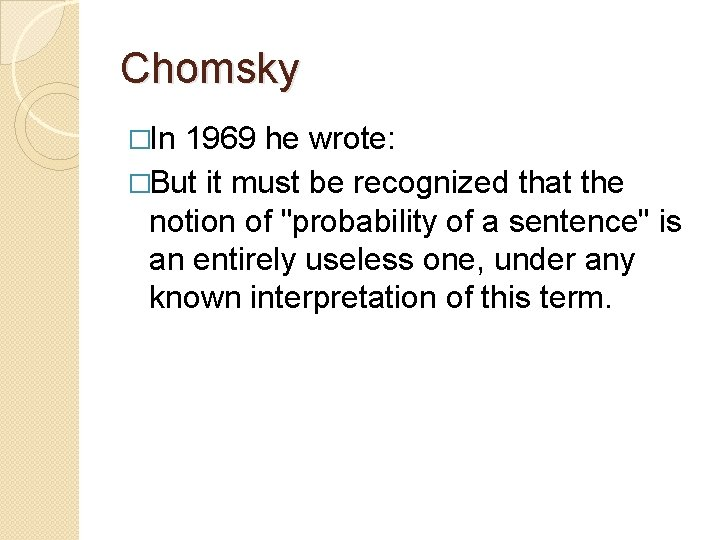Chomsky �In 1969 he wrote: �But it must be recognized that the notion of "probability of a sentence" is an entirely useless one, under any known interpretation of this term.Chomsky’s Argument �A completely new sentence must have a probability of 0, since it is an outcome that has not been seen. �Since novel sentences are in fact generated all the time, there is a contradiction.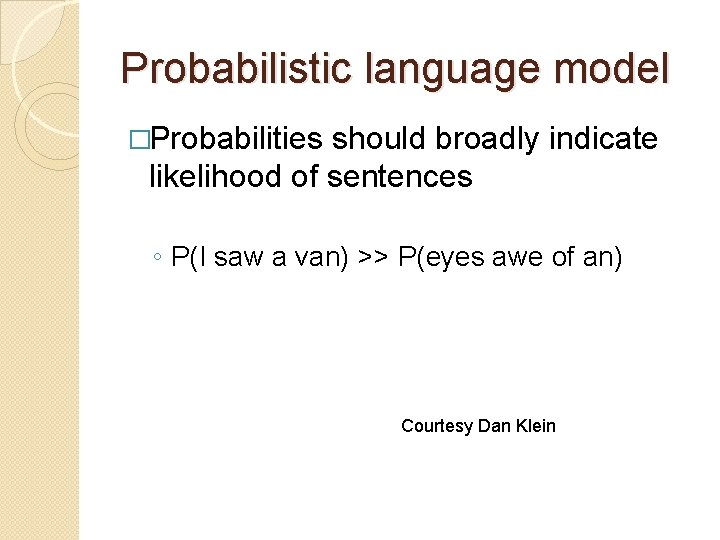Probabilistic language model �Probabilities should broadly indicate likelihood of sentences ◦ P(I saw a van) >> P(eyes awe of an) Courtesy Dan Klein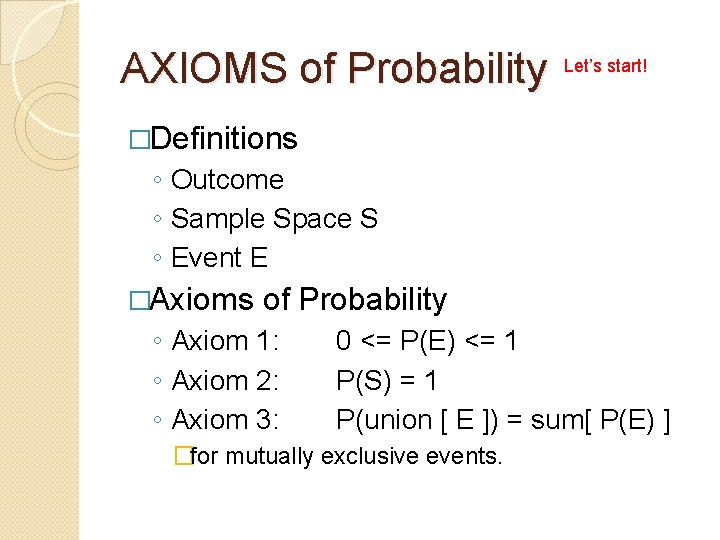AXIOMS of Probability Let’s start! �Definitions ◦ Outcome ◦ Sample Space S ◦ Event E �Axioms of Probability ◦ Axiom 1: ◦ Axiom 2: ◦ Axiom 3: 0 <= P(E) <= 1 P(S) = 1 P(union [ E ]) = sum[ P(E) ] �for mutually exclusive events.CONDITIONAL PROBABILITY ◦ P(EF) = P(E|F) * P(F) Example: Tossing 2 coins Coin 1 E Coin 2 F P(EF) 0 (tails) 0. 25 0 (tails) 1 (heads) 0. 25 1 (heads) 0 (tails) 0. 25 1 (heads) 0. 25 This is a table of P(EF). You find that no matter what E or F is, P(EF)=0. 2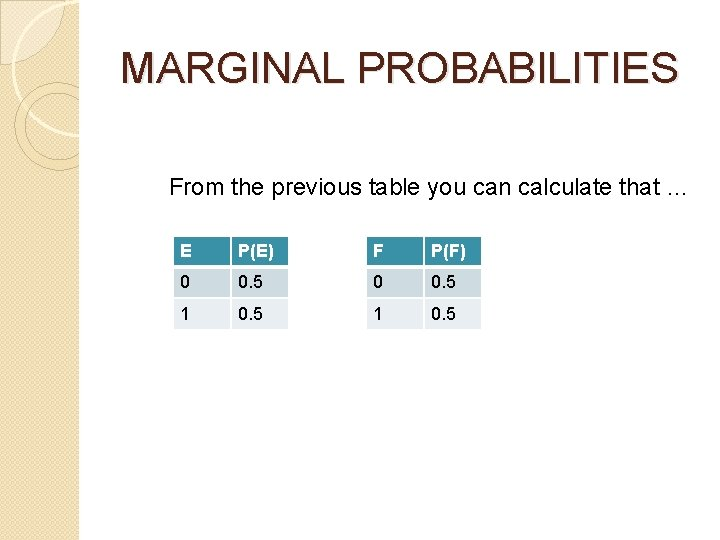MARGINAL PROBABILITIES From the previous table you can calculate that … E P(E) F P(F) 0 0. 5 1 0. 5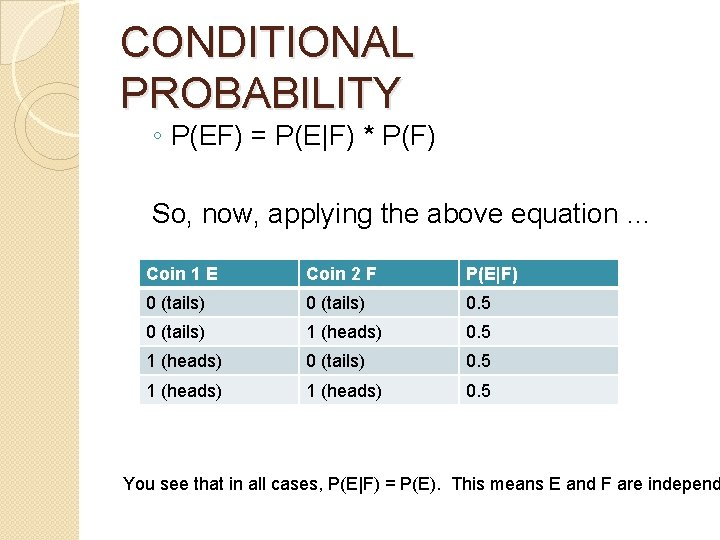CONDITIONAL PROBABILITY ◦ P(EF) = P(E|F) * P(F) So, now, applying the above equation … Coin 1 E Coin 2 F P(E|F) 0 (tails) 0. 5 0 (tails) 1 (heads) 0. 5 1 (heads) 0 (tails) 0. 5 1 (heads) 0. 5 You see that in all cases, P(E|F) = P(E). This means E and F are independ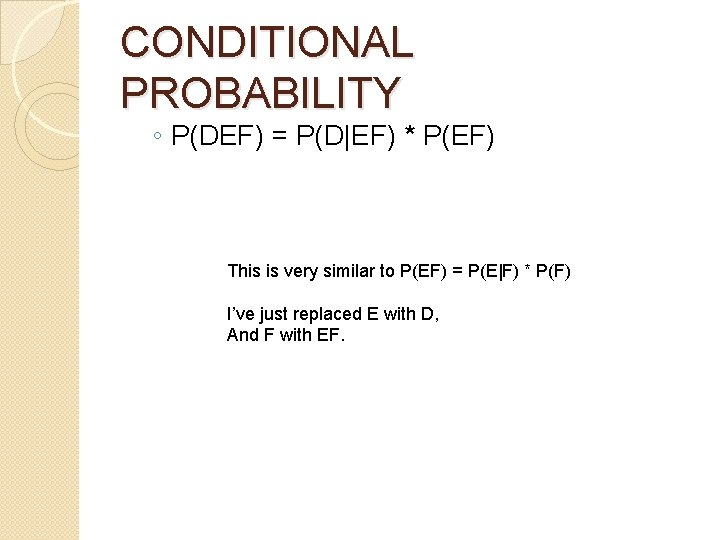CONDITIONAL PROBABILITY ◦ P(DEF) = P(D|EF) * P(EF) This is very similar to P(EF) = P(E|F) * P(F) I’ve just replaced E with D, And F with EF.CONDITIONAL PROBABILITY ◦ P(DEF) = P(D|EF) * [ P(E|F) * P(F) ] Using P(EF) = P(E|F) * P(F) P(EF )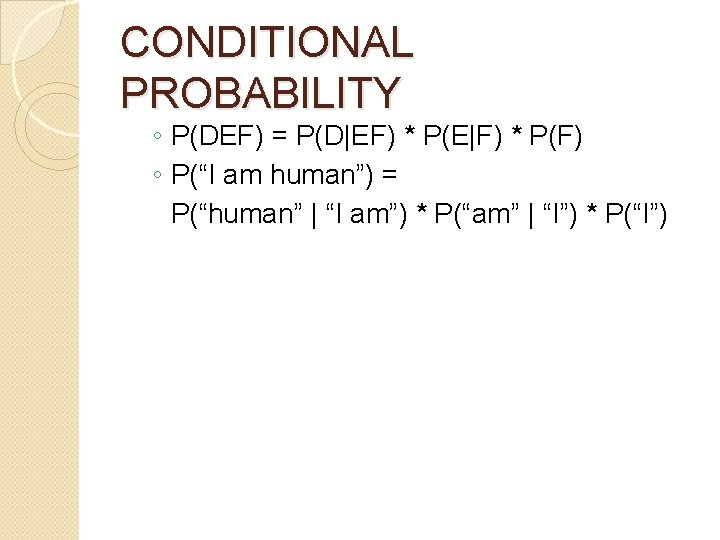CONDITIONAL PROBABILITY ◦ P(DEF) = P(D|EF) * P(E|F) * P(F) ◦ P(“I am human”) = P(“human” | “I am”) * P(“am” | “I”) * P(“I”)CONDITIONAL PROBABILITY ◦ P(DEF) = P(D|EF) * P(E|F) * P(F) ◦ P(“I am human”) = P(“human” | “I am”) * P(“am” | “I”) * P(“I”) ◦ P(“I am human”) = C(“I am human”)/C(“I am”) * C(“I am”)/C(“I”) * C(“I”)/C(“*”)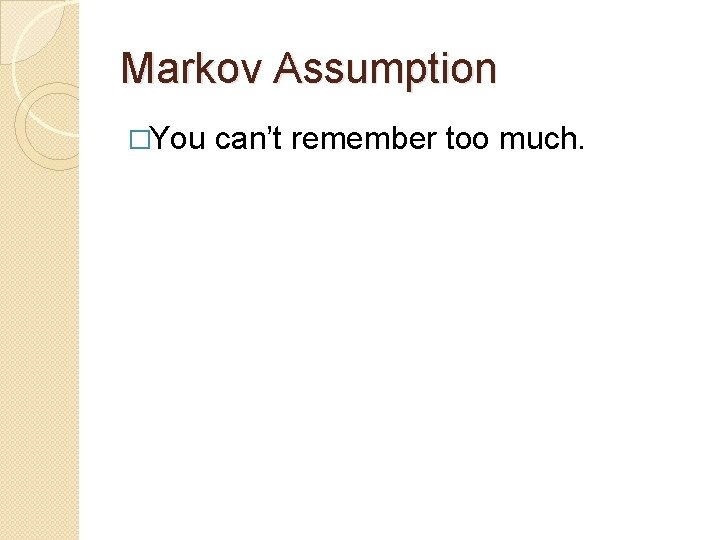Markov Assumption �You can’t remember too much.CONDITIONAL PROBABILITY ◦ P(DEF) = P(D|EF) * P(E|F) * P(F) ◦ P(“I am human”) = P(“human” | “I am”) * P(“am” | “I”) * P(“I”) ◦ P(“I am human”) = C(“I am human”)/C(“I am”) * C(“I am”)/C(“I”) * C(“I”)/C(“*”) So, you ‘forget’ the green stuff.CONDITIONAL PROBABILITY ◦ P(DEF) = P(D|EF) * P(E|F) * P(F) ◦ P(“I am human”) = P(“human” | “am”) * P(“am” | “I”) * P(“I”) ◦ P(“I am human”) = C(“am human”)/C(“am”) * C(“I am”)/C(“I”) * C(“I”)/C(“*”) Now this forgetful model can deal with unknown sentences. I’ll give you one.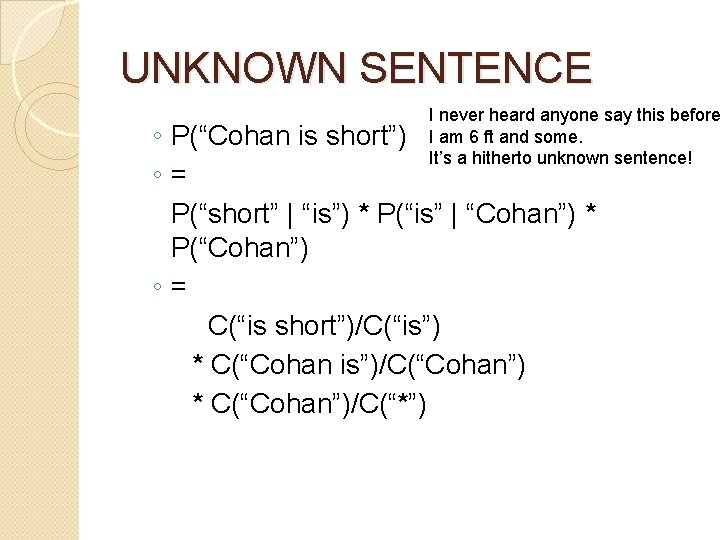UNKNOWN SENTENCE I never heard anyone say this before I am 6 ft and some. It’s a hitherto unknown sentence! ◦ P(“Cohan is short”) ◦= P(“short” | “is”) * P(“is” | “Cohan”) * P(“Cohan”) ◦= C(“is short”)/C(“is”) * C(“Cohan is”)/C(“Cohan”) * C(“Cohan”)/C(“*”)Solved! But there’s another way to solve it … and you’ll see how this has a lot to do with spell checking!Spell Checking Let’s say the word hand is mistyped Hand --- *Hamd There you have an unknown word!Spell Checking Out-of-Vocabulary Error *Hamd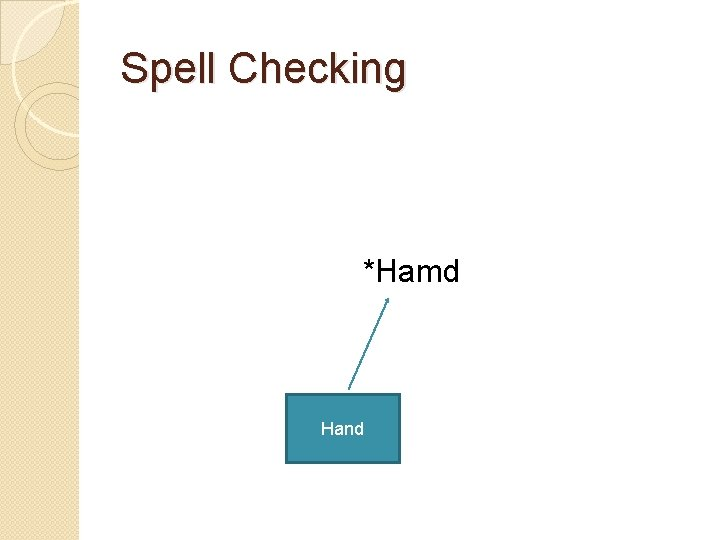Spell Checking *Hamd Hand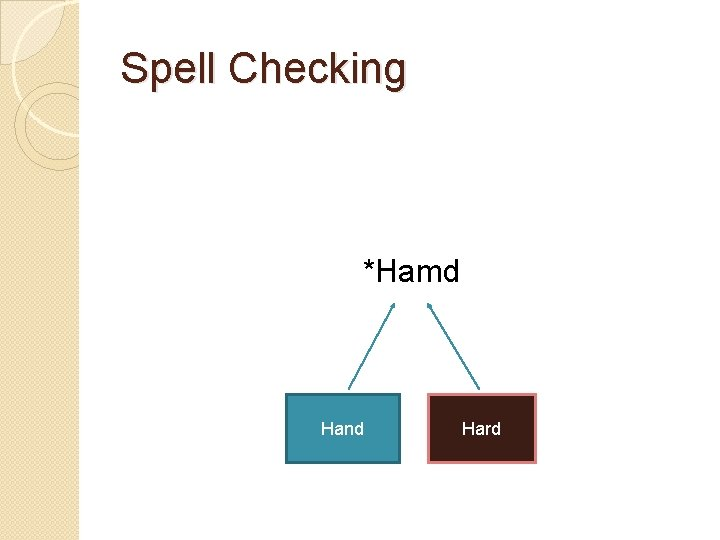Spell Checking *Hamd Hand Hard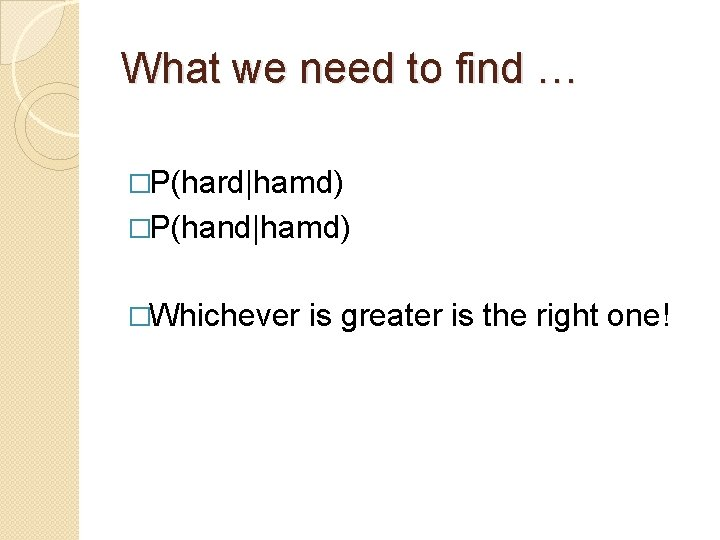What we need to find … �P(hard|hamd) �P(hand|hamd) �Whichever is greater is the right one!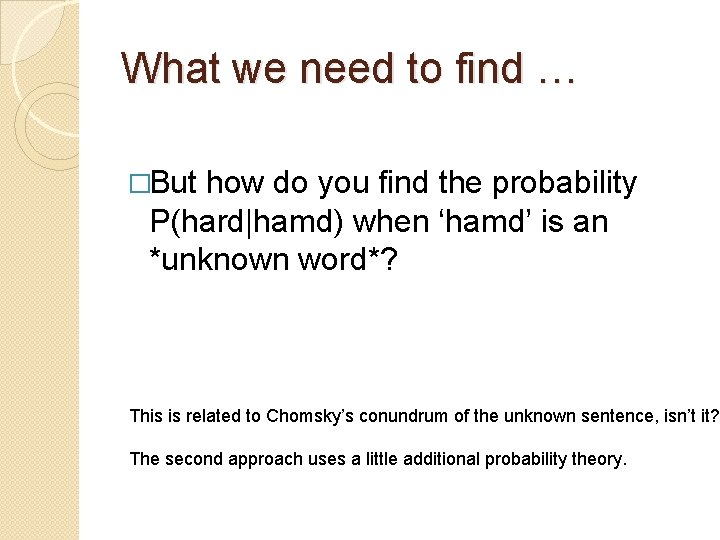What we need to find … �But how do you find the probability P(hard|hamd) when ‘hamd’ is an *unknown word*? This is related to Chomsky’s conundrum of the unknown sentence, isn’t it? The second approach uses a little additional probability theory.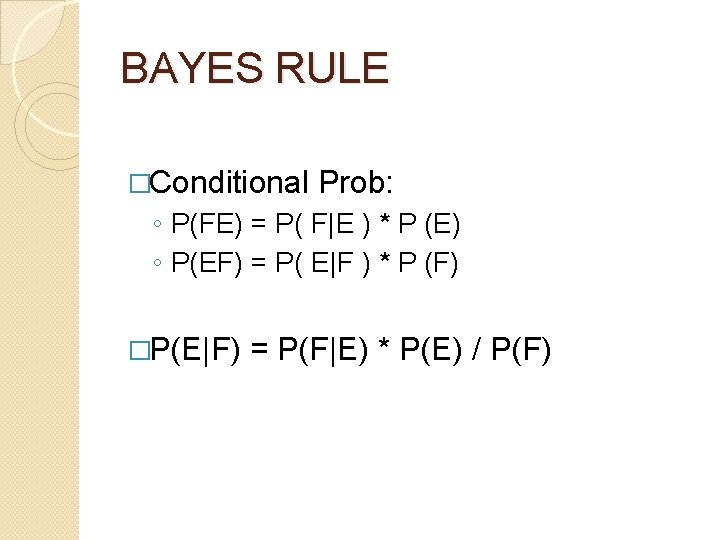BAYES RULE �Conditional Prob: ◦ P(FE) = P( F|E ) * P (E) ◦ P(EF) = P( E|F ) * P (F) �P(E|F) = P(F|E) * P(E) / P(F)BAYES RULE �P(E|F) = P(F|E) * P(E) / P(F) �P(hand|hamd) = P(hamd|hand)*P(hand)BAYES RULE �P(E|F) = P(F|E) * P(E) / P(F) �P(hand|hamd) = P(hamd|hand)*P(hand) P(hamd|hand) = P(h|h)P(a|a)P(m|n)P(d|d)Kernighan’s paper on spell correction 1990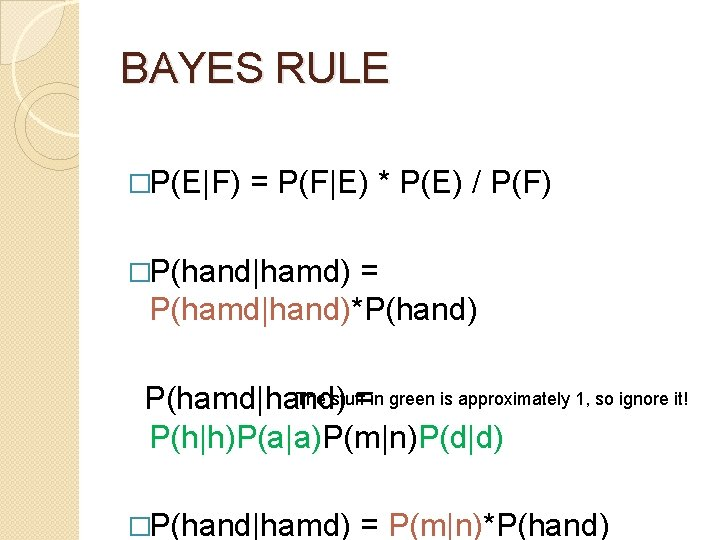BAYES RULE �P(E|F) = P(F|E) * P(E) / P(F) �P(hand|hamd) = P(hamd|hand)*P(hand) The stuff in green is approximately 1, so ignore it! P(hamd|hand) = P(h|h)P(a|a)P(m|n)P(d|d) �P(hand|hamd) = P(m|n)*P(hand)So we have what we needed to find … �P(hard|hamd) = P(m|n)*P(hand) �P(hand|hamd) = P(m|r)*P(hard) �Whichever is greater is the right one!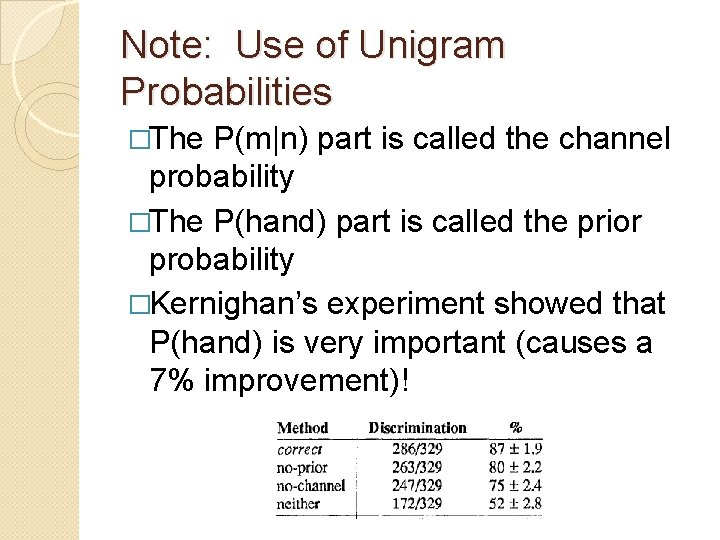Note: Use of Unigram Probabilities �The P(m|n) part is called the channel probability �The P(hand) part is called the prior probability �Kernighan’s experiment showed that P(hand) is very important (causes a 7% improvement)!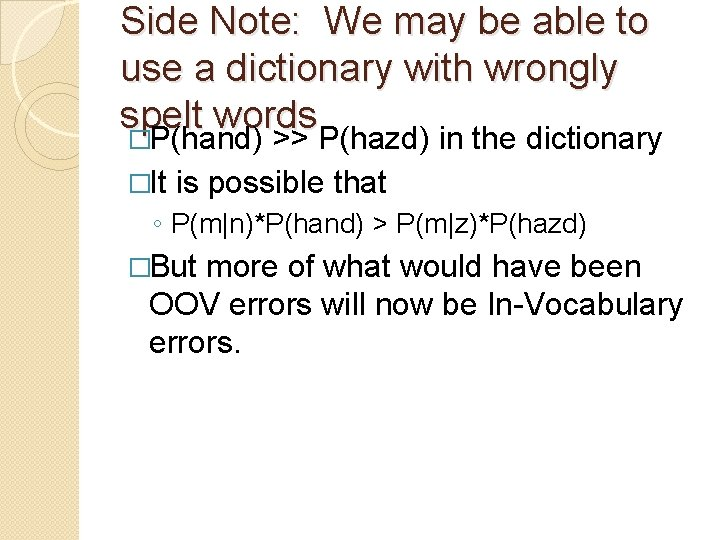Side Note: We may be able to use a dictionary with wrongly spelt words �P(hand) >> P(hazd) in the dictionary �It is possible that ◦ P(m|n)*P(hand) > P(m|z)*P(hazd) �But more of what would have been OOV errors will now be In-Vocabulary errors.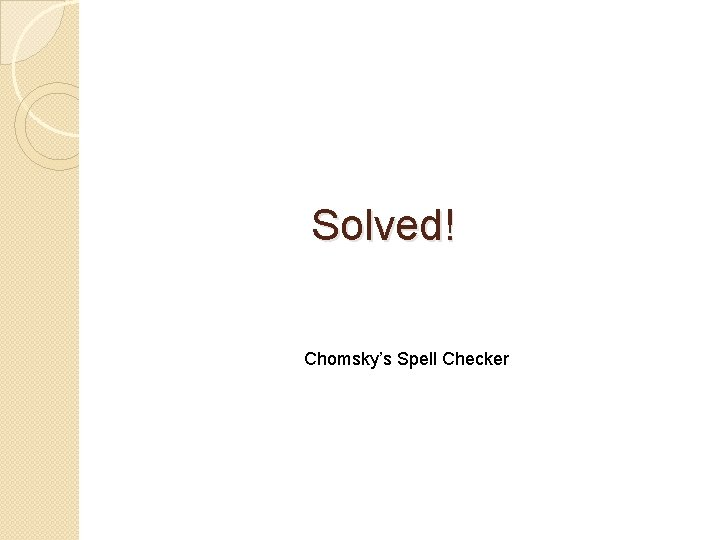Solved! Chomsky’s Spell CheckerBut there’s another interesting problem in Spell Checking … �When is an unknown word not an error?Spell Checking �How do you tell whether an unknown word is a new word or a spelling mistake? �How can you tell “Shivaswamy” is not a wrong spelling of “Shuddering” �You can use statistics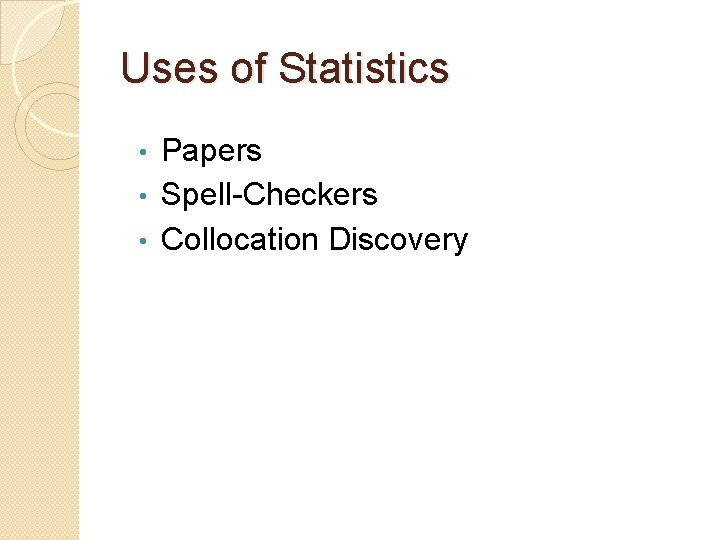Uses of Statistics Papers • Spell-Checkers • Collocation Discovery •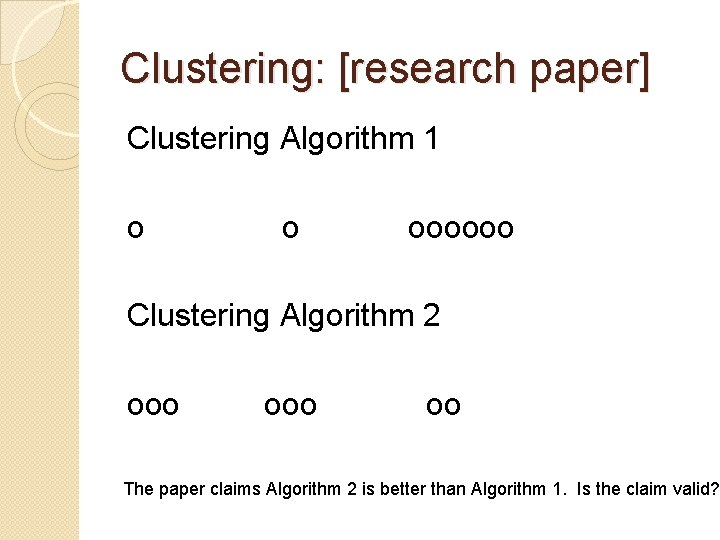Clustering: [research paper] Clustering Algorithm 1 o o oooooo Clustering Algorithm 2 ooo oo The paper claims Algorithm 2 is better than Algorithm 1. Is the claim valid?Clustering Algorithm 1 o o oooooo Clustering Algorithm 2 ooo Now, what do you think? oo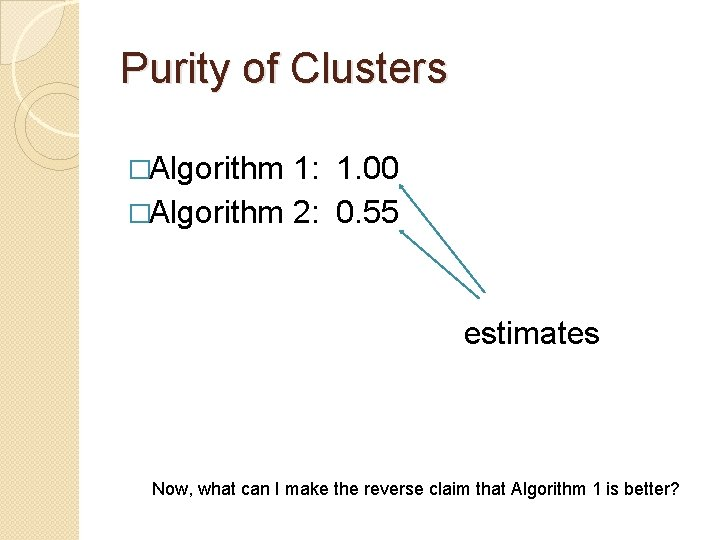Purity of Clusters �Algorithm 1: 1. 00 �Algorithm 2: 0. 55 estimates Now, what can I make the reverse claim that Algorithm 1 is better?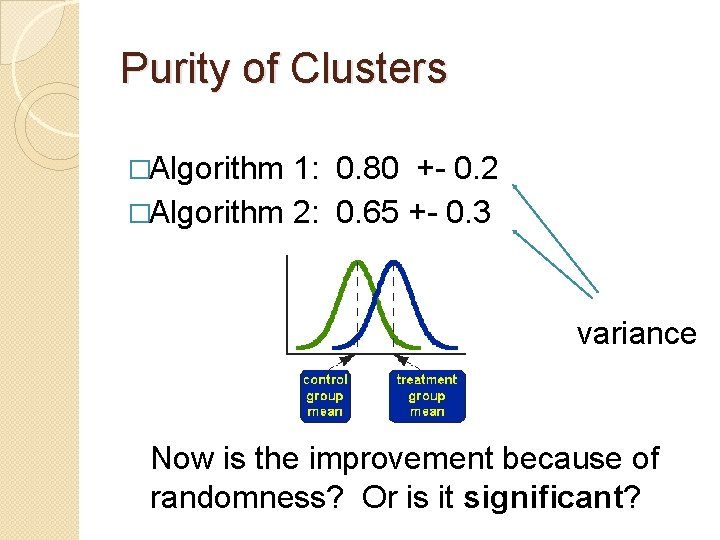Purity of Clusters �Algorithm 1: 0. 80 +- 0. 2 �Algorithm 2: 0. 65 +- 0. 3 variance Now is the improvement because of randomness? Or is it significant?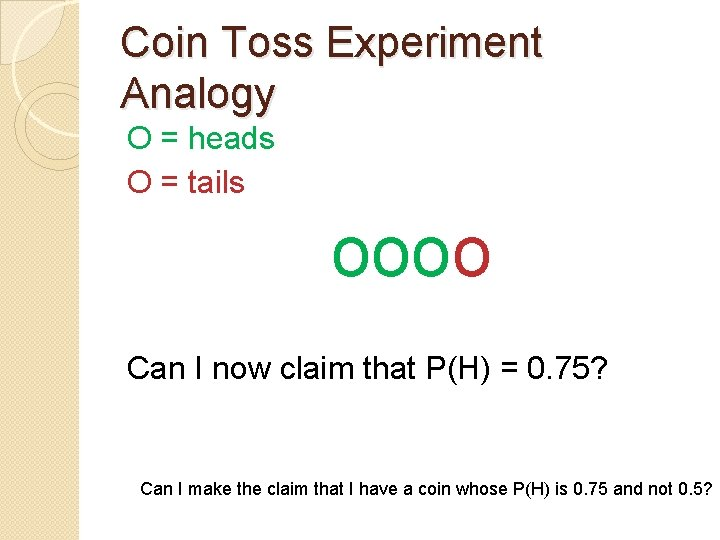Coin Toss Experiment Analogy O = heads O = tails oooo Can I now claim that P(H) = 0. 75? Can I make the claim that I have a coin whose P(H) is 0. 75 and not 0. 5?Check significance with a Ttest The t-test will show that oooo could very easily have occurred by chance on a coin with P(H) = 0. 5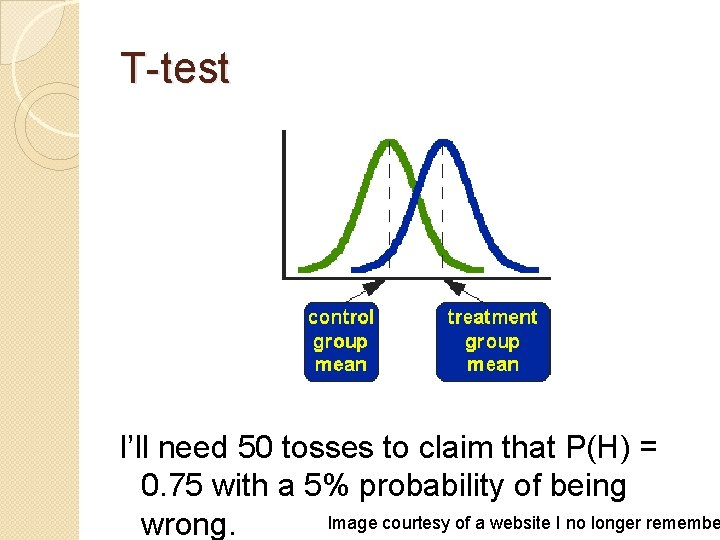T-test I’ll need 50 tosses to claim that P(H) = 0. 75 with a 5% probability of being Image courtesy of a website I no longer remembe wrong.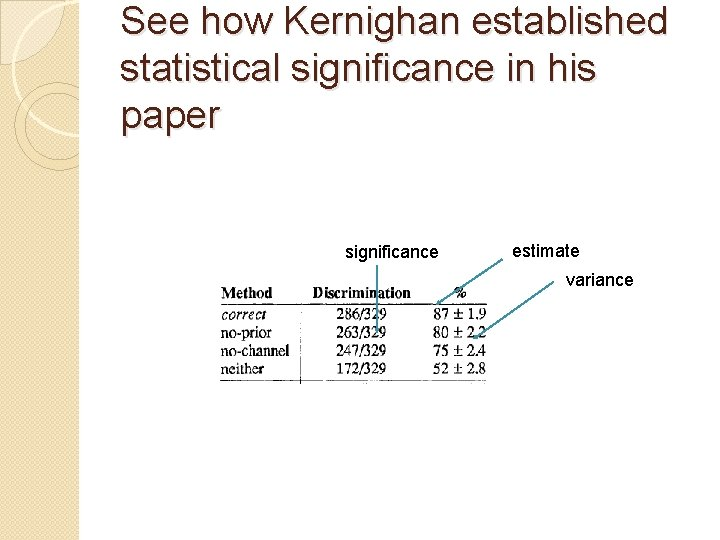See how Kernighan established statistical significance in his paper significance estimate varianceT-test You can also use the T-test in spell checking! Image courtesy of a website I no longer remembe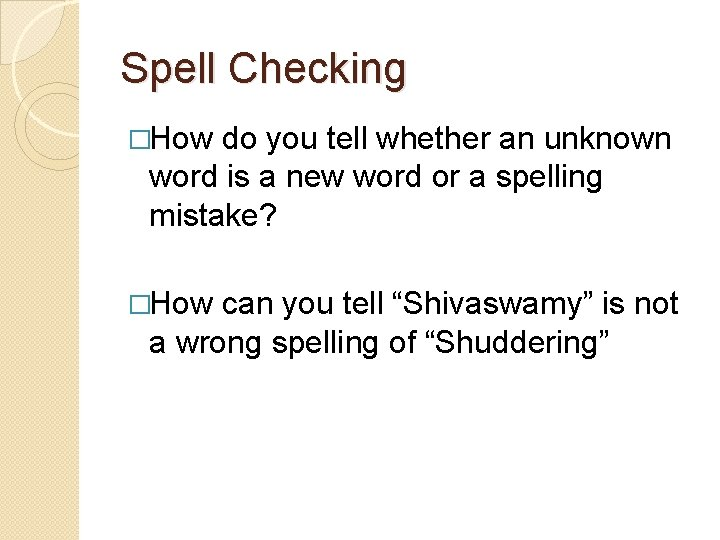Spell Checking �How do you tell whether an unknown word is a new word or a spelling mistake? �How can you tell “Shivaswamy” is not a wrong spelling of “Shuddering”T-test �Calculate the probability of “Shivaswamy” having come about by errors made on “Shuddering” … that is, 8 errors happening in 10 letters. �P(error) = 0. 8 �Say the average letter error rate is 0. 1. �Verify using the t-test that Shivaswamy is not likely to have come about from Shuddering by a 0. 1 error-per-letter process. �Use the word length (10) as n.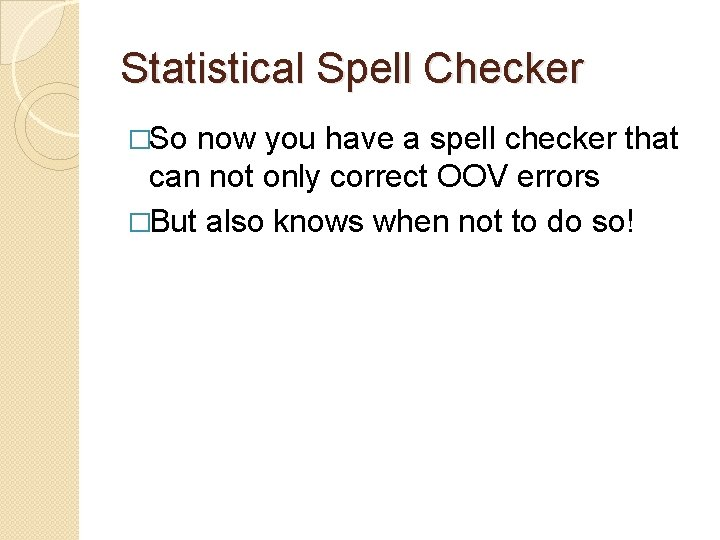Statistical Spell Checker �So now you have a spell checker that can not only correct OOV errors �But also knows when not to do so!Recap �What you have learnt in Probabilities ◦ ◦ Axioms of Probability Conditional and Joint Probabilities Independence Bayesian Inversion �Is all you will need to start reading statistical NLP papers on: ◦ ◦ Language Models Spell Checking POS tagging Machine Translation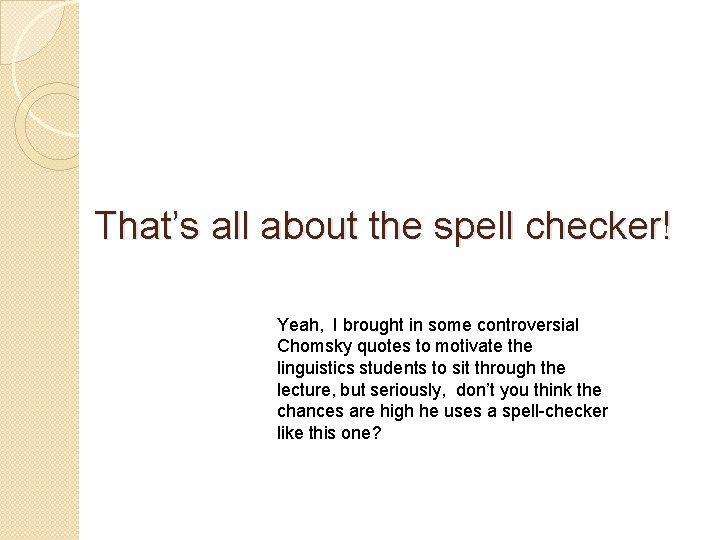That’s all about the spell checker! Yeah, I brought in some controversial Chomsky quotes to motivate the linguistics students to sit through the lecture, but seriously, don’t you think the chances are high he uses a spell-checker like this one?